﻿ 一种腐蚀增敏光纤迈克尔逊干涉型折射率传感器 A Corrosion Enhancing Optical Fiber Michelson Interference Type Refractive Index Sensor

Optoelectronics
Vol.07 No.03(2017), Article ID:22206,8 pages
10.12677/OE.2017.73014

A Corrosion Enhancing Optical Fiber Michelson Interference Type Refractive Index Sensor

Wenxian Li1,2, Qian Liu1,2, Yanhua Ma1,2, Xiaozhe Ding1,2, Lijun Li 1,2

1College of Electronic communication and Physics, Shandong University of Science and Technology, Qingdao Shandong

2Provincial Key Laboratory of mine disaster prevention and control, Qingdao Shandong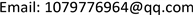Received: Sep. 7th, 2017; accepted: Sep. 22nd, 2017; published: Sep. 28th, 2017ABSTRACT

In this paper, a refractive index sensor based on Michelson interference principle is fabricated by using thin-core fiber (TCF). The sensor uses a two-stage single-mode fiber (SMF) in the middle of melting a thin-core fiber to form Michelson interference structure. The cladding mode is excited at the first fusion point and the interference occurs at the second fusion point. The experimental results show that with the increase of the intensity of the core, the increase of the refractive index causes the interference fringe to be blue-shifted. Then, in order to enhance the sensitivity of the sensor, we have corroded the thin-core fiber  . After the etching, it is found that under the low refractive index, the refractive index has a good linear relationship with the wavelength. Compared with the pre-corrosion sensor, the sensor performance which after the corrosion has been greatly improved. The experimental results show that the refractive index reaches -564.09 nm/RIU at the long wavelength in the high refractive index region of 1.42-1.4435, and the sensitivity at the second, third and fourth bands was increased by 0.9655, 0.71 and 0.7 times, respectively, compared with that before the non-etching.

Keywords:Refractive Index Sensing, Thin-Core Fiber, Michelson Interference, Corrosion

1山东科技大学，电子通信与物理学院，山东 青岛

2矿山灾害预防控制省部共建国家重点实验室培育基地，山东 青岛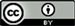1. 引言

2. 传感器的制作与原理

$I={I}_{1}+{I}_{2}+2\sqrt{{I}_{1}{I}_{2}}\mathrm{cos}\left[2\text{π}\left({n}_{co}^{eff}-{n}_{cl,j}^{eff}\right)L/\lambda \right]$ (1)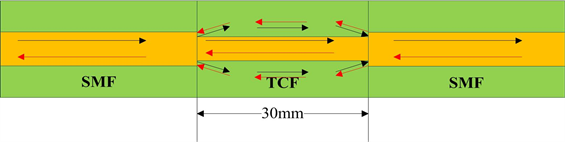Figure 1. Sensor structure and transmission light schematic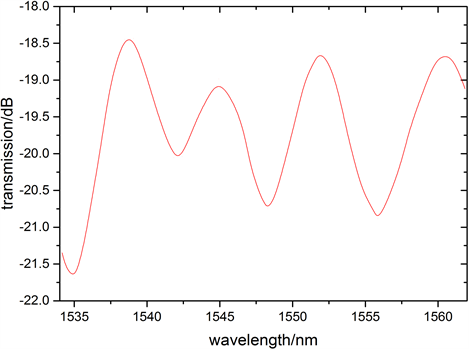Figure 2. Interference spectrum of the sensor

$\Delta \phi =2\text{π}\left({n}_{co}^{eff}-{n}_{cl,j}^{eff}\right)L/\lambda =2\text{π}\Delta {n}_{eff}L/\lambda$ (2)

(2)式中： $\Delta {n}_{eff}$ 表示纤芯模与包层模的折射率差。结合(1)式和(2)式可知，当 $\Delta \phi$$\text{π}$ 的奇数倍时即 $\Delta \phi =\left(2k+1\right)\text{π}$ 时，干涉光强达到最小，此时干涉谱中相应的干涉谷特征波长可表示为：

$\lambda =2\left({n}_{co}^{eff}-{n}_{cl,j}^{eff}\right)L/\left(2k+1\right)=2\Delta {n}_{eff}L/\left(2k+1\right)$ (3)

$\partial {\lambda }_{j}/\partial n=-\left(2L/2k+1\right)\cdot \left(\partial {n}_{cl,j}^{eff}/\partial n\right)$ (4)

3. 实验结果与数据分析

3.1. 低折射率实验

3.1.1. 腐蚀前实验

3.1.2 腐蚀后实验

3.2. 高折射率实验

3.2.1. 腐蚀前实验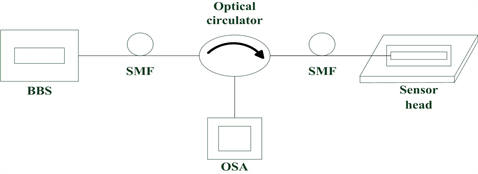Figure 3. Experimental setup diagram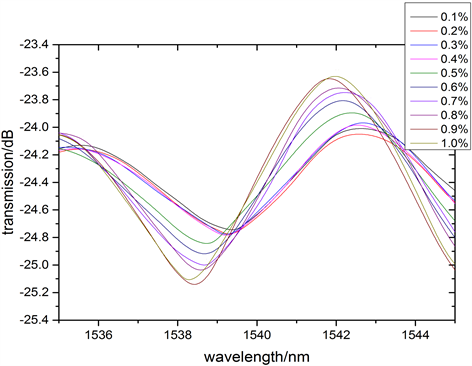Figure 4. Interference spectra of low refractive index area after etching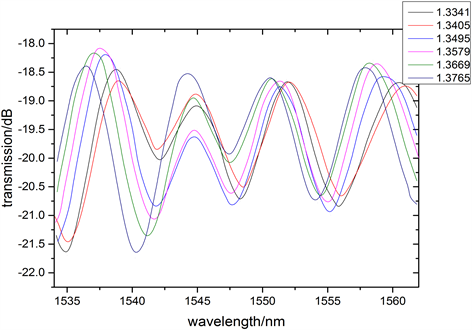Figure 5. The interference spectra of the refractive index region 1.3341~1.3765 before etching

3.2.2. 腐蚀后实验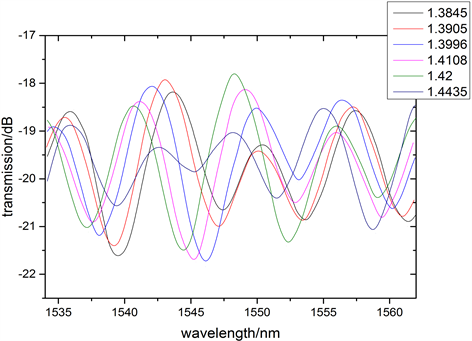Figure 6. The interference spectra of the refractive index region 1.3845~1.4435 before etching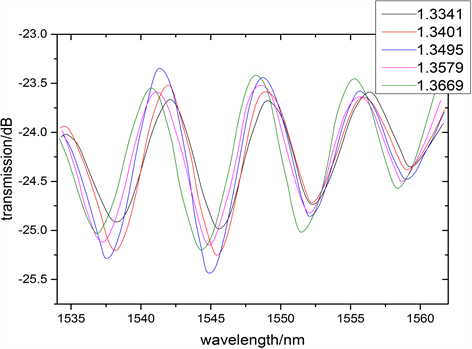Figure 7. The interference spectra of the refractive index region 1.3341~1.3669 after etching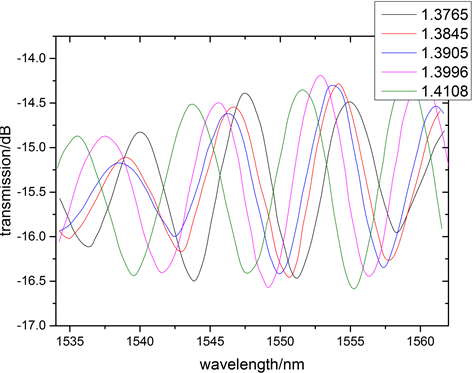Figure 8. The interference spectra of the refractive index region 1.3765~1.4108 after etching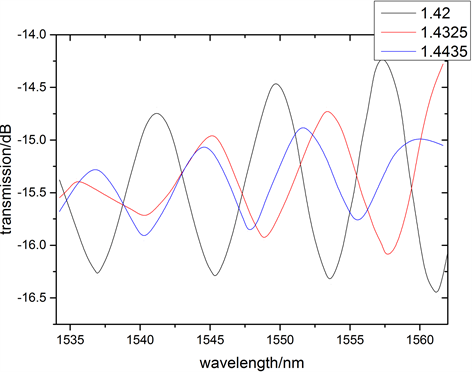Figure 9. The interference spectra of the refractive index region 1.42~1.4435 after etching

4个波谷都向短波方向漂移，且漂移量由短波向长波方向依次为6.8 nm、13.633 nm、18.6 nm和18.667 nm。

4. 结论

A Corrosion Enhancing Optical Fiber Michelson Interference Type Refractive Index Sensor[J]. 光电子, 2017, 07(03): 95-102. http://dx.doi.org/10.12677/OE.2017.73014

1. 1. Chen, J.P., Zhou, J. and Shu, L. (2013) High-Sensitivity Mach-Zehnder Interferometer Refractive Index Sensor Based on Clad-ding-Etched Fiber. Opto-Electronic Engineering, 40, 52-56.

2. 2. 白春河, 罗云瀚, 陈哲, 等. 基于侧边抛磨光纤倏逝场的折射率传感特性[J]. 光子学报, 2013, 42(10): 1182-1186.

3. 3. 张桂娜, 袁雪梅. SMS光纤结构用于液体折射率测量的特性[J]. 电子质量, 2016(6): 78-85.

4. 4. 宋海峰, 龚华平, 等. 基于波长与强度双解调的光纤温度传感器[J]. 光电子激光, 2013, 24(9): 1694-1697.

5. 5. 刘凡凡. SMS结构光纤温度传感器[D]: [硕士学位论文]. 杭州: 浙江大学, 2013.

6. 6. 苏丹. 基于保偏光纤的应变传感器研究[D]: [硕士学位论文]. 西安: 西北大学, 2015.

7. 7. 王栋远, 孙明明, 金永兴, 等. 同时测量温度和曲率的光纤传感器[J]. 光子学报, 2015, 44(11): 20-24.

8. 8. 傅海威, 闫旭, 李辉栋, 邵敏, 等. 基于纤芯失配型马赫–曾德尔光纤折射率和温度同时测量传感器研究[J]. 光学学报, 2014, 34(11): 58-63.

9. 9. 高平安, 荣强周, 孙浩, 忽满利, 等. 纤芯失配熔接的高灵敏度光纤折射率传感器[J]. 应用光学, 2013, 34(3): 542-546.

10. 10. 谢灵骁, 张信普, 李丽霞, 彭伟, 等. 基于弯曲光纤的折射率传感器研究[J]. 光电子激光, 2016, 27(3): 247-252.

11. 11. 李辉栋, 傅海威, 邵敏, 等. 基于光纤气泡和纤芯失配的Mach-Zehnder干涉液折射率传感器[J]. 物理学报, 2013, 62(2): 65-71.